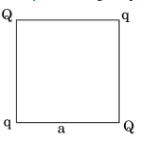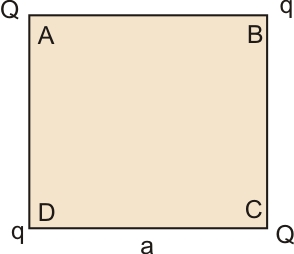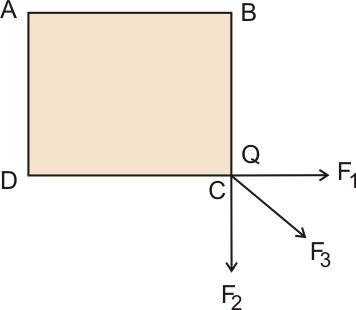# Four point charges Q, q, Q and q are placed at the corners of a square of side ‘a’ as shown in the figure.Find  the (a) resultant electric force on a charge Q, and (b) potential energy of this system.

a)Force on charge Q at C is the resultant force of all other charges.
By Coulomb's law force between two charges is,

where q1 and q2 are charges separated by distance 'r'
By applying this law, the force due to charge q is
By q at D,

By q at B,
where 'a' is the distance between charges
The distance between charge Q at C and Q atA is ,
So, by, Applying Coulomb's law, we getThe resultant of force F1 and F2 is along F3
That is ,

Now, the net force is

The direction of net force is along AC
b) The potential energy between two charges separated by a distance r is

For finding the potential energy of a system, first, find separate potential energy.
The potential energy between charges which are near corners is (separated by a)

The potential energy between charge q and q is

The potential energy between charge Q and Q is

Therefore, the net potential energy of a system is

## Related Chapters

### Preparation Products

##### Knockout NEET July 2020

An exhaustive E-learning program for the complete preparation of NEET..

₹ 15999/- ₹ 6999/-
##### Rank Booster NEET 2020

This course will help student to be better prepared and study in the right direction for NEET..

₹ 9999/- ₹ 4999/-
##### Knockout JEE Main July 2020

An exhaustive E-learning program for the complete preparation of JEE Main..

₹ 12999/- ₹ 6999/-
##### Test Series NEET July 2020

Take chapter-wise, subject-wise and Complete syllabus mock tests and get in depth analysis of your test..

₹ 4999/- ₹ 2999/-